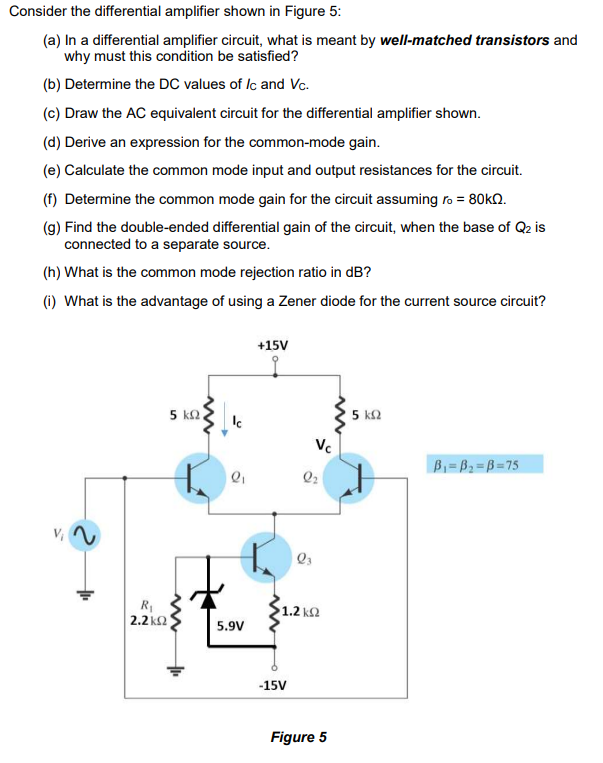# Question Consider the differential amplifier shown in Figure 5: (a) In a differential amplifier circuit, what is meant by well-matched transistors and why must this condition be satisfied? (b) Determine the DC values of lc and Vc. (c) Draw the AC equivalent circuit for the differential amplifier shown. (d) Derive an expression for the common-mode gain. (e) Calculate the common mode input and output resistances for the circuit. (f) Determine the common mode gain for the circuit assuming ro = 80k12. (9) Find the double-ended differential gain of the circuit, when the base of Q2 is connected to a separate source. (h) What is the common mode rejection ratio in dB? (1) What is the advantage of using a Zener diode for the current source circuit? +15V 5 ΚΩ, IC 5 5k2 Vc B=B=B=75 O 02 Vz Q R 2.2KΩ 1.2 ΚΩ 5.9V -15V Figure 5ZJKWPQ The Asker · Electrical EngineeringTranscribed Image Text: Consider the differential amplifier shown in Figure 5: (a) In a differential amplifier circuit, what is meant by well-matched transistors and why must this condition be satisfied? (b) Determine the DC values of lc and Vc. (c) Draw the AC equivalent circuit for the differential amplifier shown. (d) Derive an expression for the common-mode gain. (e) Calculate the common mode input and output resistances for the circuit. (f) Determine the common mode gain for the circuit assuming ro = 80k12. (9) Find the double-ended differential gain of the circuit, when the base of Q2 is connected to a separate source. (h) What is the common mode rejection ratio in dB? (1) What is the advantage of using a Zener diode for the current source circuit? +15V 5 ΚΩ, IC 5 5k2 Vc B=B=B=75 O 02 Vz Q R 2.2KΩ 1.2 ΚΩ 5.9V -15V Figure 5
More
Transcribed Image Text: Consider the differential amplifier shown in Figure 5: (a) In a differential amplifier circuit, what is meant by well-matched transistors and why must this condition be satisfied? (b) Determine the DC values of lc and Vc. (c) Draw the AC equivalent circuit for the differential amplifier shown. (d) Derive an expression for the common-mode gain. (e) Calculate the common mode input and output resistances for the circuit. (f) Determine the common mode gain for the circuit assuming ro = 80k12. (9) Find the double-ended differential gain of the circuit, when the base of Q2 is connected to a separate source. (h) What is the common mode rejection ratio in dB? (1) What is the advantage of using a Zener diode for the current source circuit? +15V 5 ΚΩ, IC 5 5k2 Vc B=B=B=75 O 02 Vz Q R 2.2KΩ 1.2 ΚΩ 5.9V -15V Figure 5